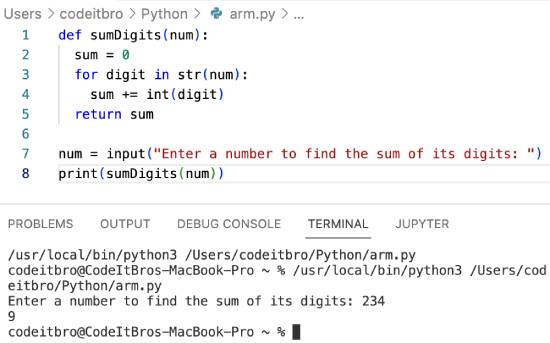# Python Program to Find Sum of Digits [5 Methods]

94

In this Python program, we will see how to find the sum of digits. For example, if the input is 123, the output should be 6. To calculate the sum of digits, we will cover five different methods:

• Using int() and str() methods
• Using the sum() method
• Find the sum of digits in Python using for loop
• Sum of digits in Python using while loop
• Find the sum of digits in Python using recursion

## Method 1: Using int() and str() methods

In Python, you can use the str() function to convert integers to strings and the int() function to convert string numbers to integers.

So, this Python program will first convert the input number to a string. After that, we will iterate through this string and add its integer literals by converting them to int data type.

Code:

```def sumDigits(num):
sum = 0
for digit in str(num):
sum += int(digit)
return sum

num = input("Enter a number to find the sum of its digits: ")
print(sumDigits(num))```

Output:## Method 2: Using sum() method

Here we will use the str() to convert a number to a string, strip the string, and convert it to a list of numbers using the strip() and map() methods, respectively. Then use the getSum(num) method to find the sum.

Source Code:

```def getSum(num):
convertStringNum = str(num)
sumDigit = list(map(int, convertStringNum.strip()))
return sum(sumDigit)

num = input("Enter the number to find the sum of digits: ")
print(getSum(num))```

Output:

## Method 3: Python program to find the sum of digits using for loop

In this Python program, we will see how to find the sum of digits using for loop. First, we will input the number from the user and initialize a variable sumDigits to store the sum of digits.

After that, we will use the for loop to iterate through the input number, a string. During each iteration, we will store the sum of digits after converting the string literal to int.

In the final step, we will print the sumDigits variable to display the sum of all digits.

Also ReadWhat Does The Python Percent Sign Mean?

Code:

```num = input("Enter a number to find the sum of digits using for loop: ")
sumDigits = 0
for n in num:
sumDigits = sumDigits + int(n)
print(sumDigits)```

## Method 4: Python program to find the sum of digits of a number using while loop

Code:

```num = int(input("Enter any number to find sum of its digits: "))
sumDigits = 0

while(num > 0):
Reminder = num % 10
sumDigits = sumDigits + Reminder
num = num //10

print("The sum of the digits = %d" %sumDigits)```

Also ReadHow to Create an Empty Array In Python

## Method 5: Python program to find the sum of digits using recursion

Code:

```def sumDigits(n):
if n< 10:
return n
else:
return n%10 + sumDigits(n/10)

number = int(input("Enter a number to find the sum of digits: "))

sum = sumDigits(number)

print("Sum of digit of number %d is %d." % (number, sum)```

### Summary

In this Python program, we shared how to find the sum of digits using various methods:

• int() and str() methods
• sum() function
• Sum of digits using for() and while() loops
• Find the sum of digits using recursion

Other Python articles you must read: